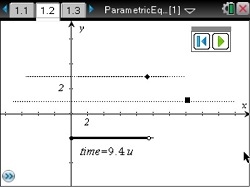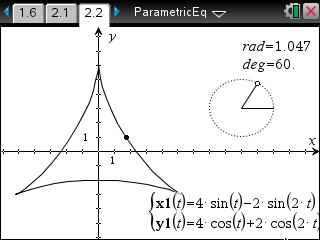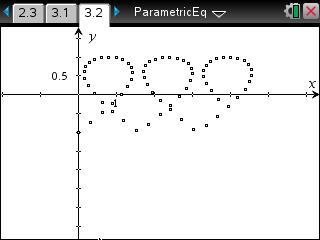# Activities

••• ##### Subject Area

• Math: Calculus: Parametric Equations and Polar Coordinates

• ##### Author9-12

20 Minutes

• ##### Device
• TI-Nspire™ CAS
• ##### Software

TI-Nspire™ CAS

3.0

## Parametric Races & Slopes

#### Activity Overview

Students will study the motion of two points and use calculus to determine the velocity and acceleration. The slope of parametric equations will be determined.

#### Key Steps

•Students will investigate parametric equations. Students will study the motion of two points and use calculus to determine the velocity and acceleration. Self-check multiple-choice questions are used to help students check their understanding.

•Next, students will explore a graph that is not a function, but can be graphed with parametric equations. They will grab and move the point on the circle to change the ‘time’ that corresponds to an angle in this example.

Students will learn about the vertical and horizontal slope of the tangent.

•As an extension, students will try to create a graph from parametric equations that matches the some given points. For whatever interesting graph they make, students will find the value of t for the vertical and horizontal tangents.